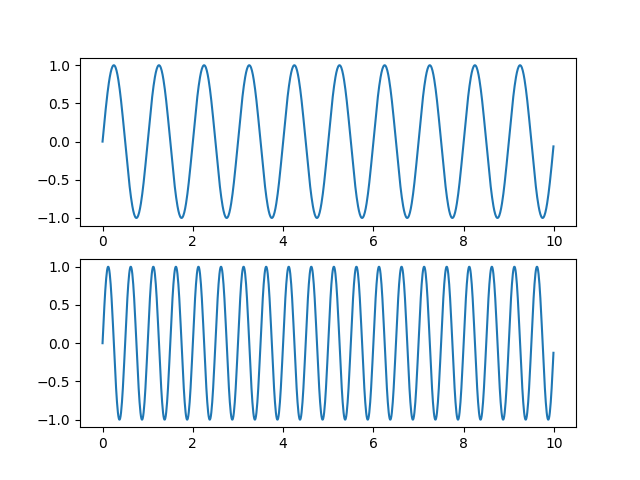Travis-CI:# Sharing axis limits and views¶

It’s common to make two or more plots which share an axis, e.g., two subplots with time as a common axis. When you pan and zoom around on one, you want the other to move around with you. To facilitate this, matplotlib Axes support a `sharex` and `sharey` attribute. When you create a `subplot()` or `axes()` instance, you can pass in a keyword indicating what axes you want to share with```import numpy as np
import matplotlib.pyplot as plt

t = np.arange(0, 10, 0.01)

ax1 = plt.subplot(211)

ax1.plot(t, np.sin(2*np.pi*t))

ax2 = plt.subplot(212, sharex=ax1)

ax2.plot(t, np.sin(4*np.pi*t))
```

Total running time of the script: ( 0 minutes 0.035 seconds)

Gallery generated by Sphinx-Gallery